﻿ Blackboard Inscribed With Scientific Formulas And Calculations In Physics And Mathematics. Can Illustrate Scientific Stock Vector - Illustration of drawn, algebra: 112976864

# Blackboard inscribed with scientific formulas and calculations in physics and mathematics. Can illustrate scientific.

## Blackboard inscribed with scientific formulas and calculations in physics and mathematics. Can illustrate scientific topics to quantum mechanics and any scientific

Designers Also Selected These Stock Illustrations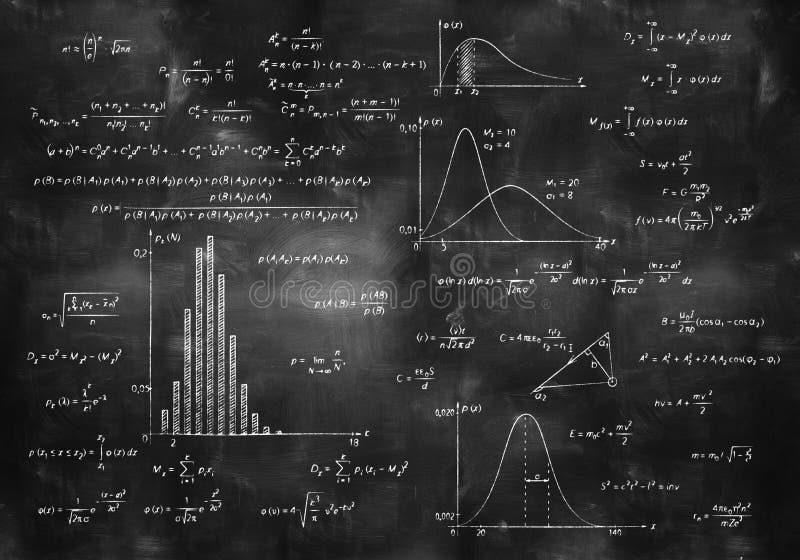Math physics formulas on chalkboardMetatron Cube Gold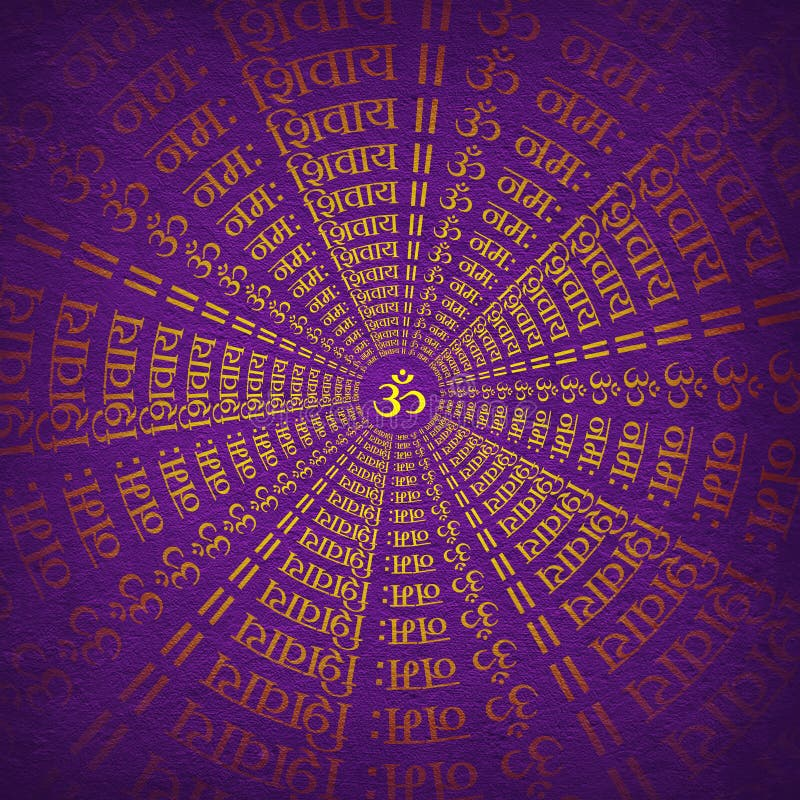Devotional Background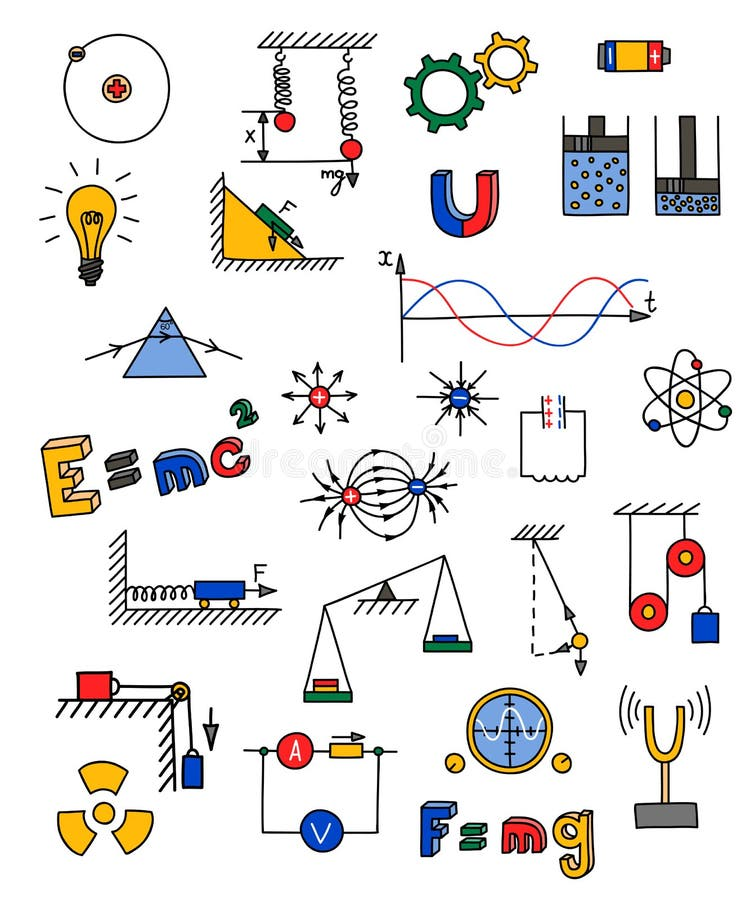Physics iconDeer and tiger head tattoo. psychedelic, zentangle style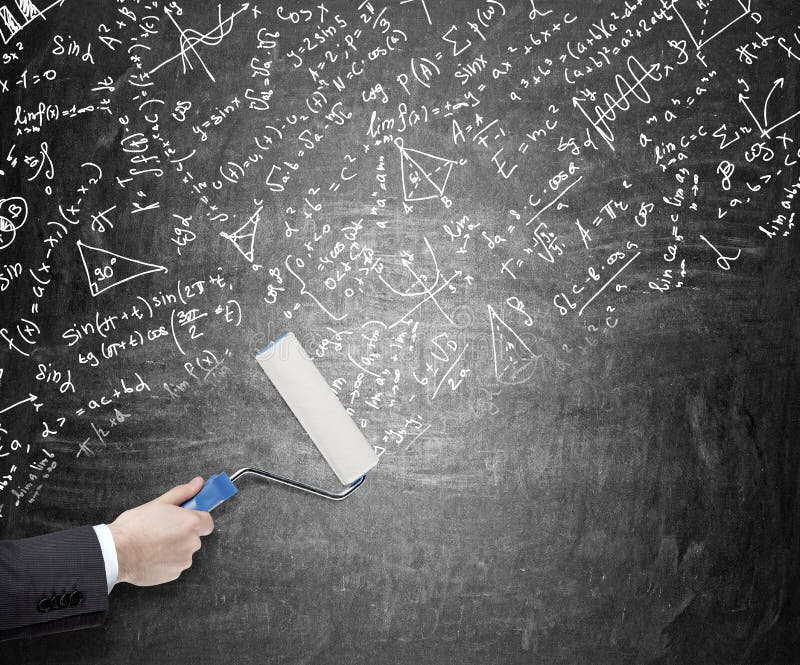Calculations on the blackboard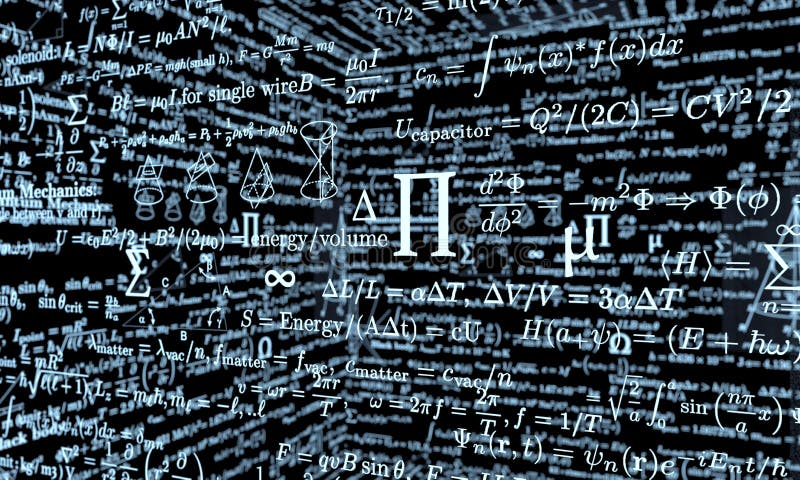Black mathematics board with formulas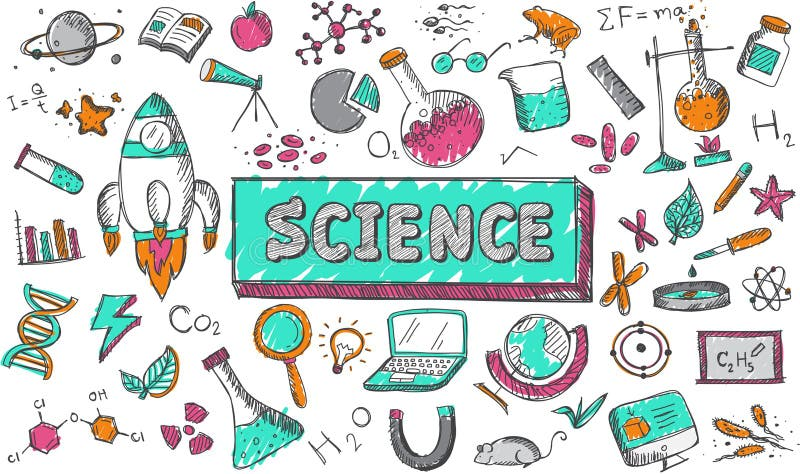Science chemistry physics biology astronomy education subjectNano physics science illusttration model concept.
More similar stock illustrations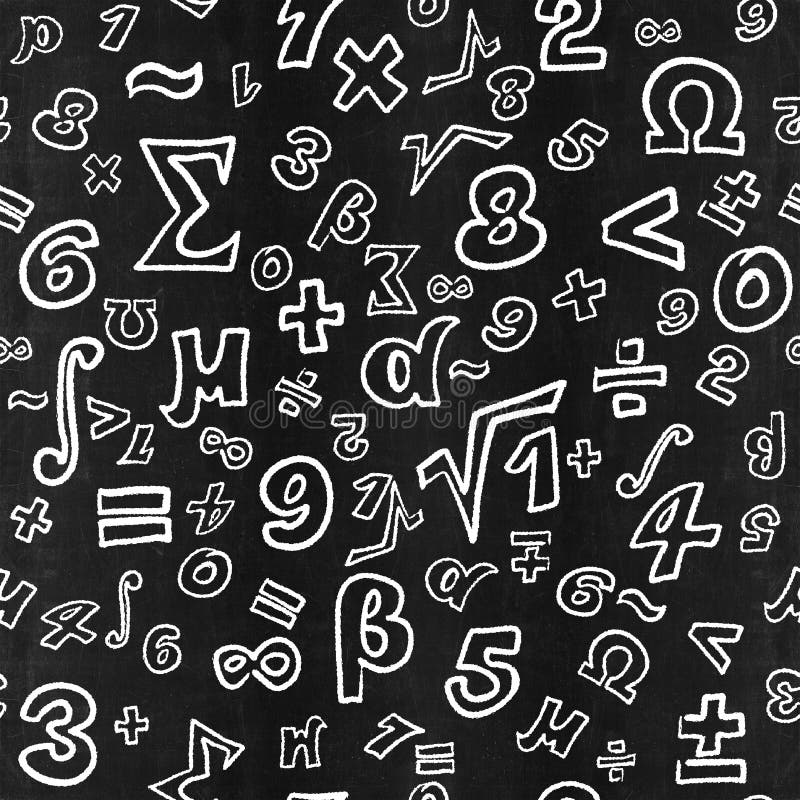Blackboard inscribed by white chalk with mathematic symbolsIllustration with mountains, firs and beautiful sky inscribed in a circle. Chalk on a blackboard.Chemistry Lesson in Elementary School Vector Flat.
More stock photos from Sergii Pal' portfolio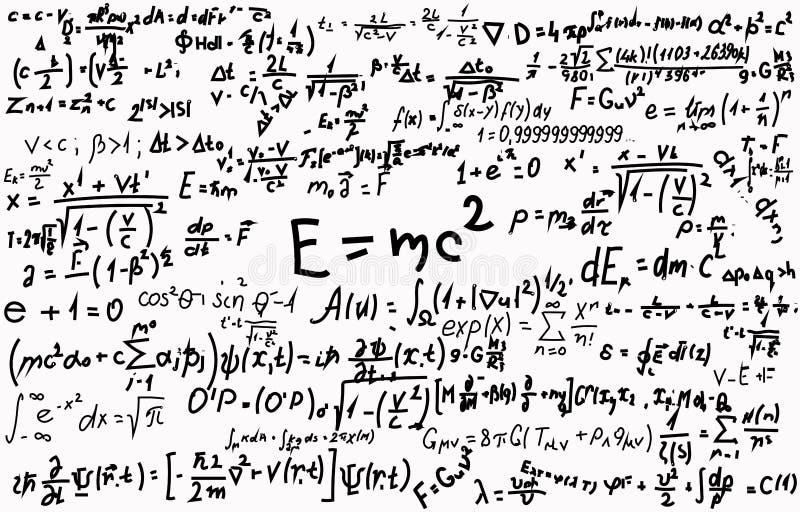Blackboard inscribed with scientific formulas and calculations in physics and mathematics. Can illustrate scientific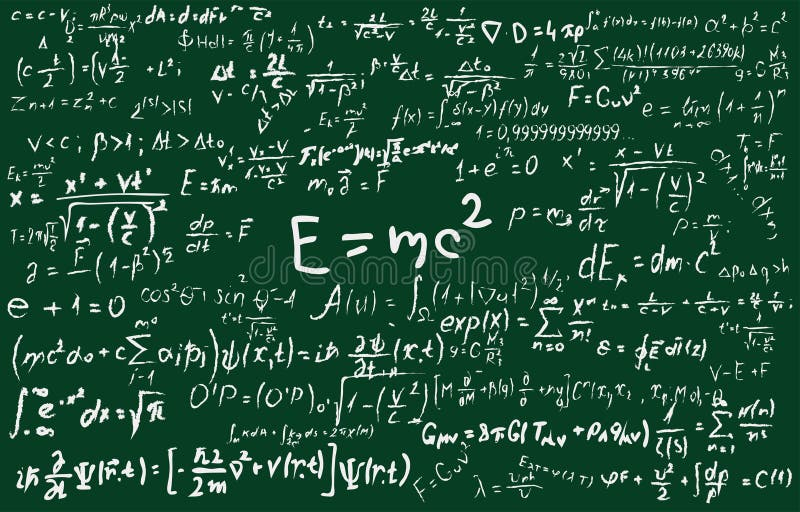Blackboard inscribed with scientific formulas and calculations in physics and mathematics. Can illustrate scientific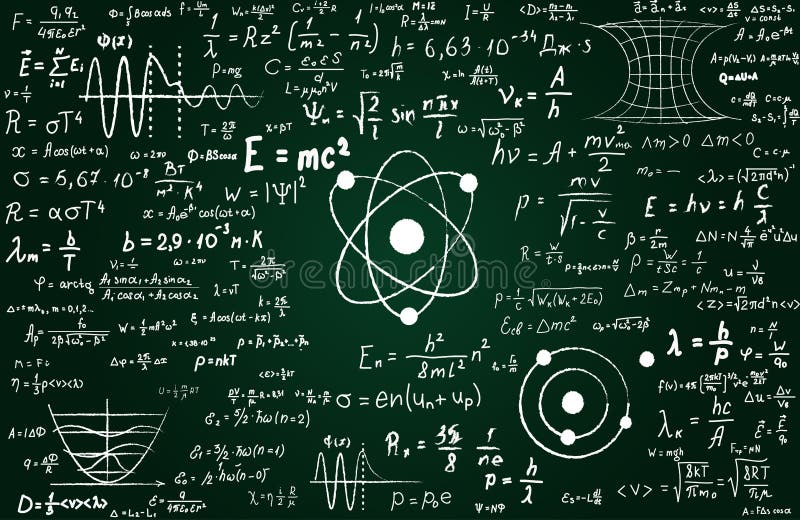Blackboard inscribed with scientific formulas and calculations in physics and mathematics. Can illustrate scientific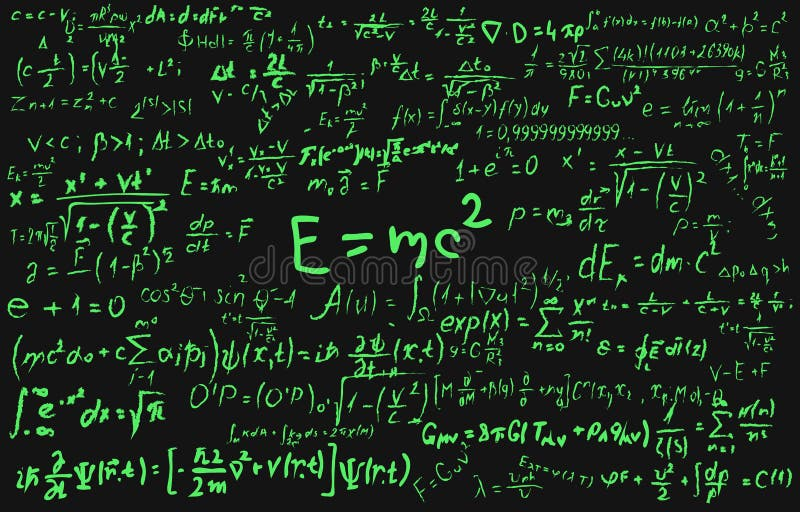Blackboard inscribed with scientific formulas and calculations in physics and mathematics. Can illustrate scientific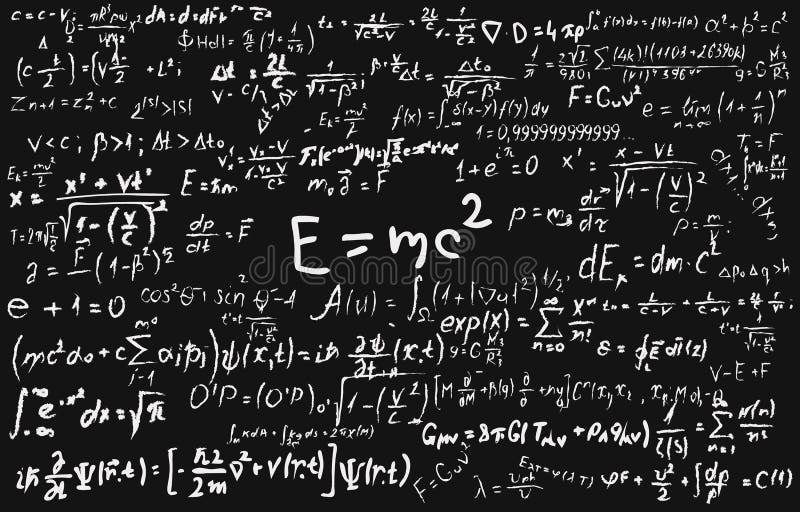Blackboard inscribed with scientific formulas and calculations in physics and mathematics.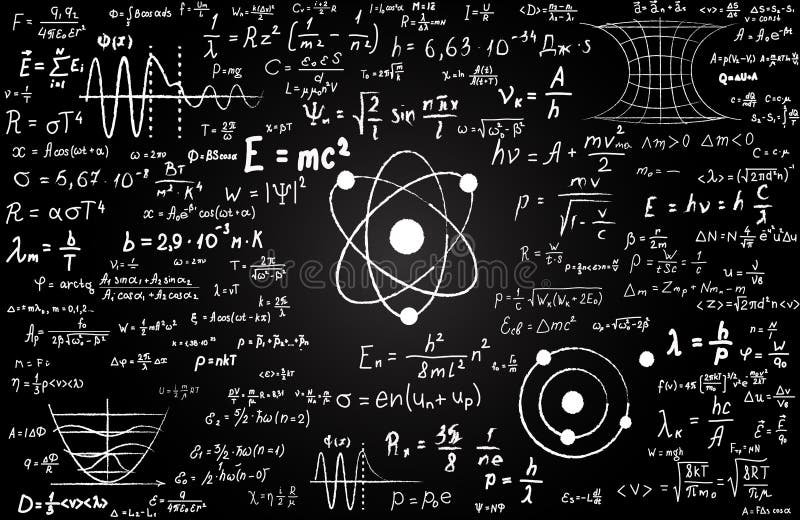Blackboard inscribed with scientific formulas and calculations in physics and mathematics. Can illustrate scientific
Related categories